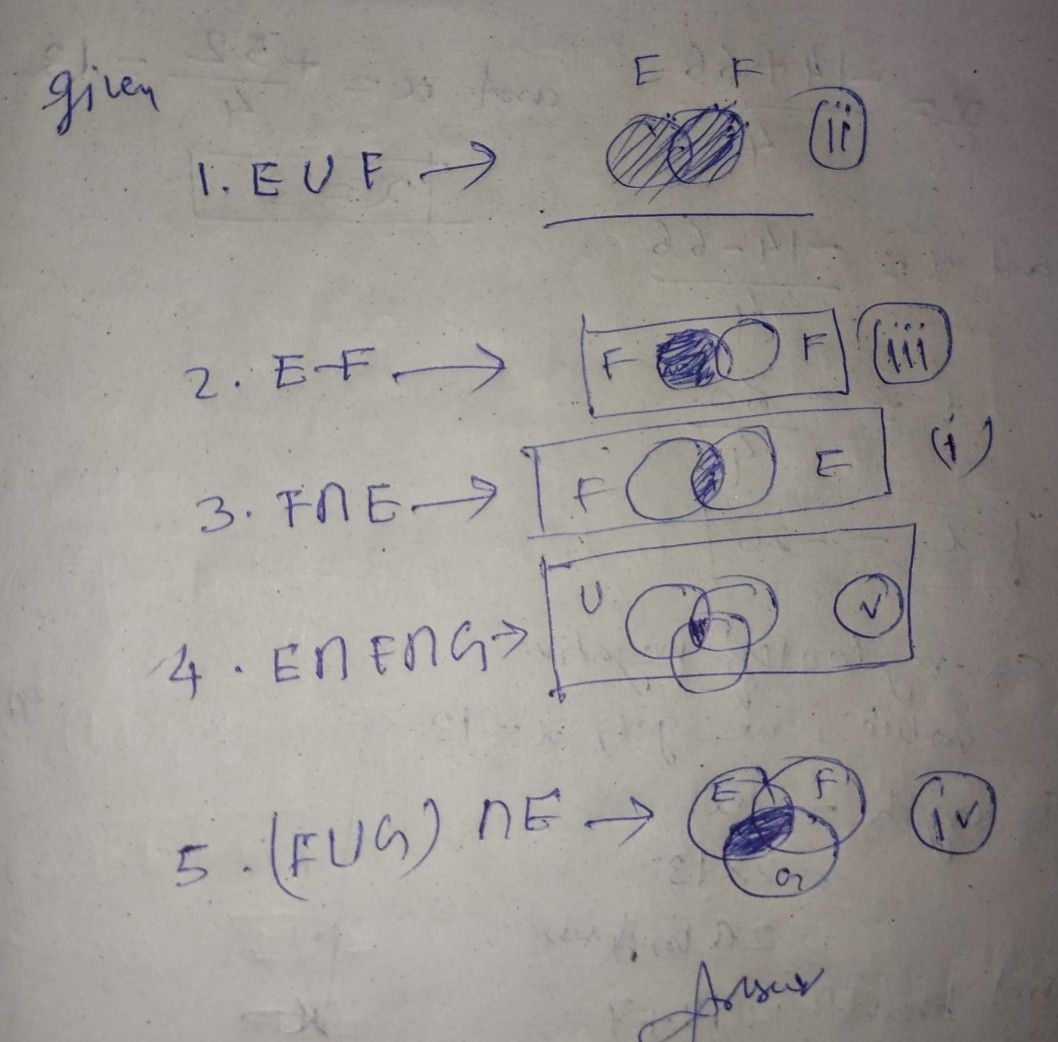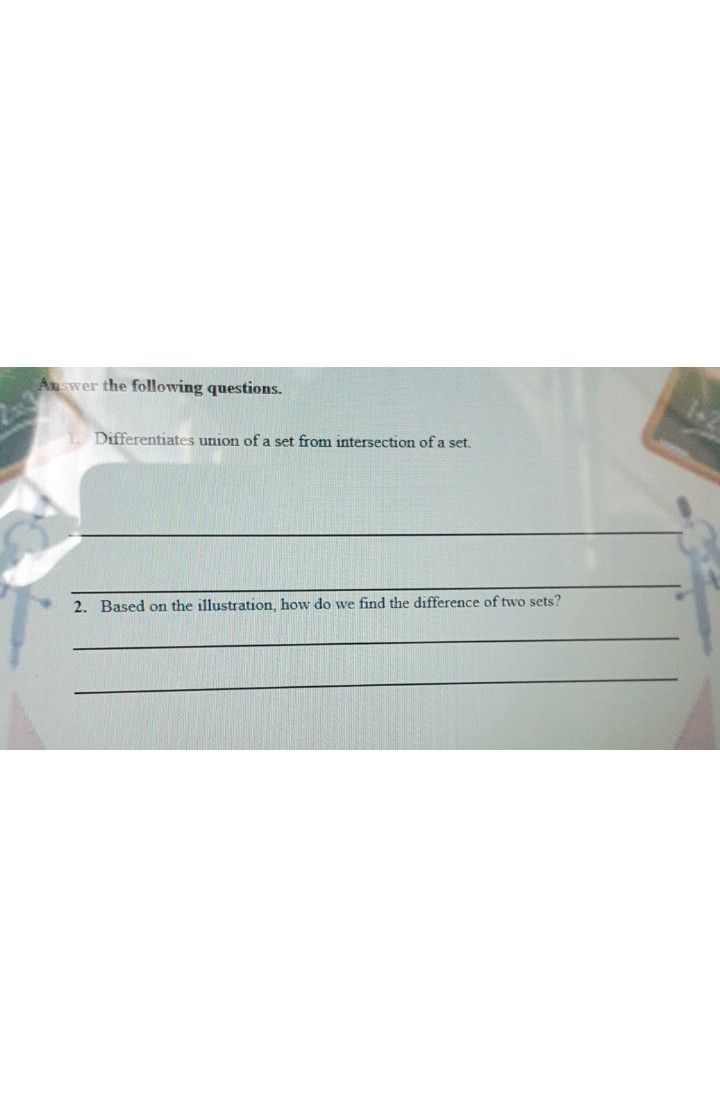Symbol
ProblemACTIVITY $4:$ "MATCH BOX" $D1RF\left(11ON:$ Match the given sets operation notation with the diagram. Write your answer on the space provided. $-$ $①$ $1$ $E∪F$ $y$ $2$ $E-P$ $3$ $P∩P$ $\bar{\dfrac {①} {\bar{O0} }}$ $4$ $E∩P∩G$ +2-3 $5,1$ $\left(P∪G\right)∩E$